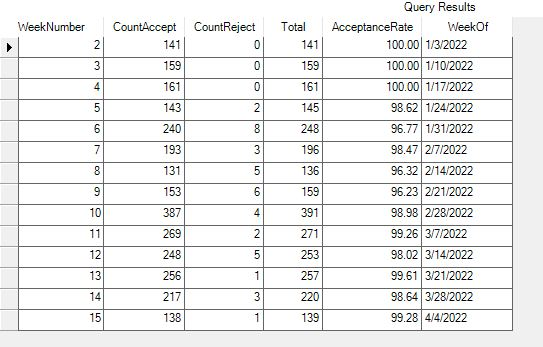# How to Join Two Subqueries based on date?

Good afternoon,
I have a BAQ called Final Acceptance Rate per week. The goal is to show the number of times a job/part/op is rejected due to scrap reasons, compared to the total number of good job/part/ops, for each week. Our Operation 900 is the final quality sign-off operation. The important thing to note here is that we are counting instances of job/part/op, not individual counts of bad parts.

For example, Job 12345, Asm 0, Op 900. There are 50 parts in laborQty, and 5 parts in ScrapQty with reason code 01. In this case, I want to see that there is 1 job/part/op. Not 5.

Over the course of a year I want a list showing week number, the accepted count of job/part/ops, and the rejected count of job/op/parts. From this I can calculate an acceptance rate for each job/part/op.

The problem I has is that I can’t join the accepted sub query with the rejected subquery. I would like to join them on Payroll date, but I need a base table that has all the dates in it to link them together. Right now I am using laborDtl again to link both accepted and rejected based on that payroll date. I think this is giving me bad results.

Is there a built-in table that I can use to just return a list of dates? Then I can link the subqueries to that table and show the totals for each week.

I understand this is a confusing request. I have attached my example BAQ for your review. I think this is very close to what I need. I appreciate any suggestions!
Nate
AcceptanceRate.baq (52.0 KB)

Note: I keep using job/part/op, when really it is job/assembly part/operation. To make sure we capture the assembly level parts.

Run them as two separate queries* and then UNION ALL them together. Take that subquery and do your sorting and summing.

• One query for accepted with zeros in rejected and the other for rejected and zero for the accepted.
1 Like

Once I union them, I need to pull the date from somewhere to populate the week number. I was pulling this from the labordtl.payrolldate that was linking the two subqueries together. Now that I unioned them, I have to choose the date from either scrap or accepted. Attached the updated based on your suggestion. I think I did what you said.AcceptanceRate.baq (48.4 KB)

A mockup of what you’re after would help me out. My 2021.2 instance imported the BAQ but complains about a bad SQL statement.

I have updated the file below to show what my original query looked like when it was (almost) working. I say almost, because it returns results in the correct format, but the counts are not what I expect.
Here is the mockup and the query that returned it.AcceptanceRate.baq (79.2 KB)

WeekNumber, CountAccept, CountReject, Total, and WeekOf.

For each LaborDtl record, calculate each of these. CountAccept would be zero or the number accepted. CountReject wouild be zero or the number rejected. Total is total, and calculate the WeekNumber and WeekOf for EACH record.

Create a new subquery that pulls this one in and breaks on WeekNumber and WeekOf and subtotals the other three.

Now create a new subquery (top) that pulls in the above one and calculate your Acceptance rate.

That would give you the above. You mention op in the first post but I didn’t see it in the example.

1 Like

I have something that is very close. Thank you! Below is the SQL code. My AcceptanceRate calculation is not coming out correctly. The total calculations all work fine. But I am getting and acceptance rate of 1 when there are weeks with no rejects, and 0 when there are weeks with any rejects. Instead it should calculate the percentage. Is there some trick I am missing?

``````select
[Mid].[Calculated_Week] as [Calculated_Week],
[Mid].[Calculated_Accepted] as [Calculated_Accepted],
[Mid].[Calculated_Rejected] as [Calculated_Rejected],
(Mid.Calculated_Accepted/ Mid.Calculated_Total) as [Calculated_AcceptanceRate]
from  (select
[Joiner].[Calculated_Week] as [Calculated_Week],
(sum(Joiner.Calculated_AccCount)) as [Calculated_Accepted],
(sum(Joiner.Calculated_RejCount)) as [Calculated_Rejected],
(Accepted+ Rejected) as [Calculated_Total]
from  (select
(datepart(wk, LaborDtl2.PayrollDate)) as [Calculated_Week],
[LaborDtl2].[ScrapReasonCode] as [LaborDtl2_ScrapReasonCode],
(iif(LaborDtl2.LaborQty >0,1,0)) as [Calculated_AccCount],
(iif(LaborDtl2.ScrapQty>0,1,0)) as [Calculated_RejCount]
from Erp.LaborDtl as LaborDtl2
where (LaborDtl2.OprSeq = 900  and LaborDtl2.PayrollDate >= '1/1/2022'))  as Joiner
group by [Joiner].[Calculated_Week])  as Mid
``````

I just had to cast the values I was dividing as floats!
I simplified the query down to this, which gives excellent results.
Thank you for the help!

``````select
[Joiner].[Calculated_Week] as [Calculated_Week],
(sum(Joiner.Calculated_AccCount)) as [Calculated_Accepted],
(sum(Joiner.Calculated_RejCount)) as [Calculated_Rejected],
(Accepted+ Rejected) as [Calculated_Total],
(cast(Accepted as float)/ cast(Total as float)) as [Calculated_Rate]
from  (select
(datepart(wk, LaborDtl2.PayrollDate)) as [Calculated_Week],
[LaborDtl2].[ScrapReasonCode] as [LaborDtl2_ScrapReasonCode],
(iif(LaborDtl2.LaborQty >0,1,0)) as [Calculated_AccCount],
(iif(LaborDtl2.ScrapQty>0,1,0)) as [Calculated_RejCount]
from Erp.LaborDtl as LaborDtl2
where (LaborDtl2.OprSeq = 900  and LaborDtl2.PayrollDate >= '1/1/2022'))  as Joiner
group by [Joiner].[Calculated_Week]
``````

AcceptanceRateNew.baq (53.5 KB)

1 Like